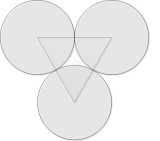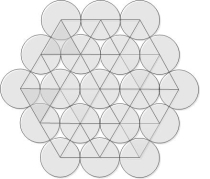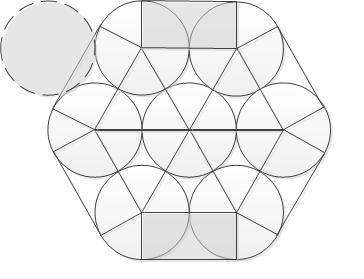# Counting pipes from the end face

The approach to the calculation of the number of nested circles of smaller radius at a known length of the describing circle of a larger radius. Created by user request

Long story short - we have a bundle of tubes tied with something. We can measure the length of the "rope". The radius of a single tube too. It's necessary to determine the number of tubes without bluntly counting them.

It obvious that we can't calculate the exact number of tubes - there are too many factors.

So we can't do without counting but the task itself is quite interesting. We can make a calculation for some ideal situation then in reality we will have no more pipes than we calculated.

Calculator making the estimation from above is situated below. And the article about it is situated below the calculator.#### Counting the number of packed circles

Digits after the decimal point: 2
The approximate total area

The area of a single circle

Estimated useful area

Number of circles (upper estimate)

The ideal case is when the tubes are lying evenly, circumference is normal etc. In general, some simplification of the problem, allowing to apply geometric knowledge and mathematical calculation :)

By the way, the estimation from above can be acquired by several methods.

For example, here is the simplest estimation from above:

1. Find the area of a circle by the length of this circle

2. Find cross-section area by the radius of the tube

3. Divide the total cross-sectional area in the cross-sectional area of one pipe

Obviously, that will be an estimation from above - you can't fit more tubes than it's possible in this circle. But this estimation won't be exact because the tubes are not too close to each other, but with gaps, and a part of the total cross-sectional area consumed by holes between the tubes. See. imageIt is necessary to take into account these losses and make an assessment of the number of tubes more accurate.For starters let's deal with the area of the gap between the pipes. For this, we consider a triangle whose vertices are formed by the centers of adjoining circles. Each side is obviously equal to twice the radius, and Heron's formula for its area is . The area is composed of useful space occupied by the three sectors (from each circle), and the hole. This sector obviously has an 180 degrees angle, and, therefore, the area of the three sectors is equal to half the area of the circle .
Thus, the ratio of useful area to the total area of the triangle is equal to
The most remarkable feature of this conclusion is that this ratio does not depend on the radius.Move on. As you can see from the figure, "loosely" packed circle can be represented as "tightly" packed triangles with a hole in the middle. Thus, with the total area of the whole bundle and, considering that it is a bundle of triangles - from the relationship derived above, you can find how many useful area in this bundle - and then divide the useful space by the area of a single circle, thus having another approximation of the tube bundle from above.

The attentive reader might say - And what about the loss of area on the edge of the bundle? Visually, they are more than the losses within the bundle. This is true. But! Firstly, this does not negate the fact that we get estimation from above - as the estimation from above, it remains true - because if the loss of the area on the borders is larger, a little bit less pipes will fit than we estimated. Secondly, how large are this losses? Can they be estimated? I will do it now.So, tightly packed bundle (by the way, the thing that the densest packing is an option, in which each circle is surrounded by six others, proved mathematically) can be represented as packed triangles and packed rectangles, plus one circle formed by the folds.

Losses of area in the rectangles, indeed, are more. Applying the same reasoning, we find that the ratio of useful and common area . The value is constant again, and can be compared - the usable area in the rectangle is less in times.

That is, the total area of the bundle is filled triangles with the ratio of usable area , rectangles with the ratio of usable areaand one more "useful" circle.Thus, the total effective area, on the basis of which it is possible to find the number of tubes in the bundle is composed of

Honestly, I was too lazy to think about how to find the total area of the triangle and the total area of the rectangles, but it seems clear that with increasing bundle radius the number of rectangles increases in proportion to the length of the circle, and also to the radius, but the number of triangles increases in proportion to the area of a circle, which means to the square of the radius - that is faster.
It follows that, for sufficiently large (in comparison with a radius of a single circle) total bundle radius of a rectangular component can be neglected, or, more precisely, to consider its loss equal to losses of triangular component, and then the beam effective area will be equal to
,and the number of tubes per bundle, respectively , which can be safely rounded to the next highest. All the same estimate from above.

Let us recall that we are talking about a large bundle, as in the small (see. the latest picture) encircling the "rope" is not close in shape to a circle, the greater the bundle compared to a single circle, the closer its shape to one big circle - that is a simplification.

I was promised to recalculate the pipes and compare the practice with the theory - now I'm waiting

URL copiado para a área de transferência